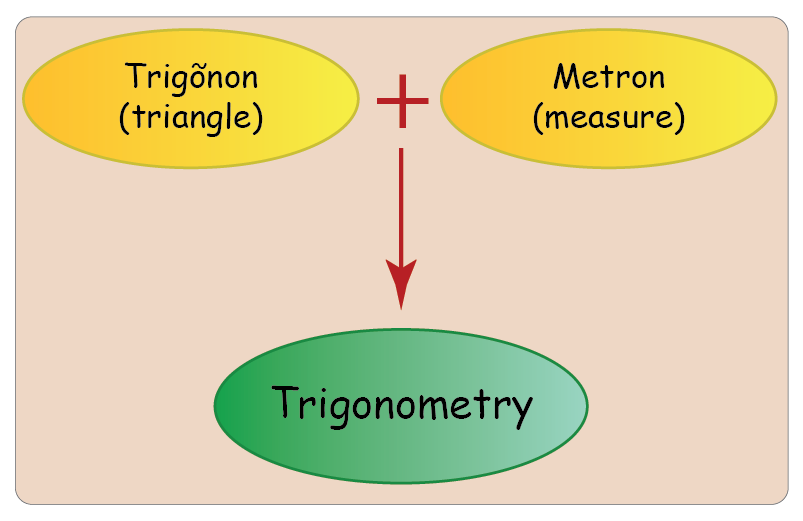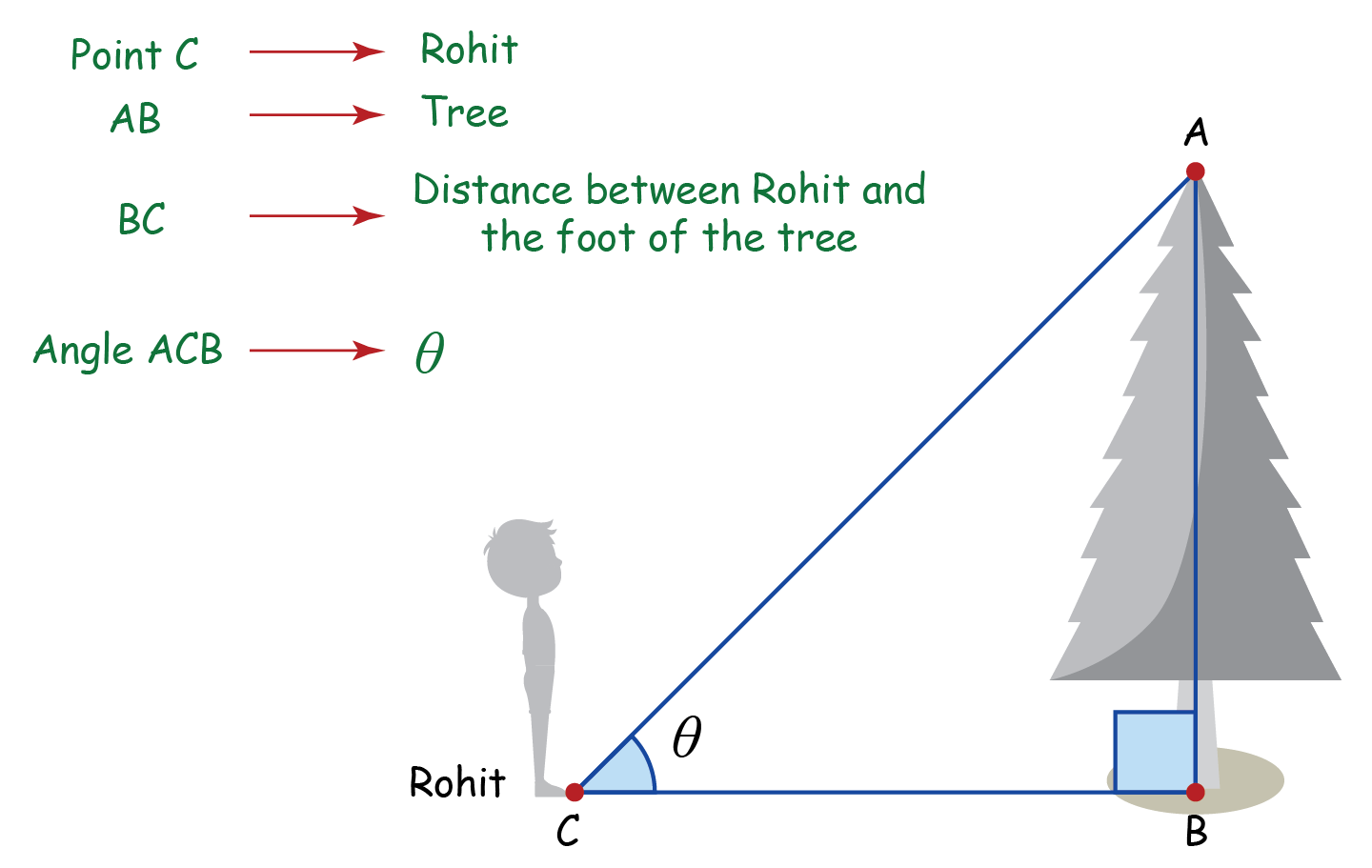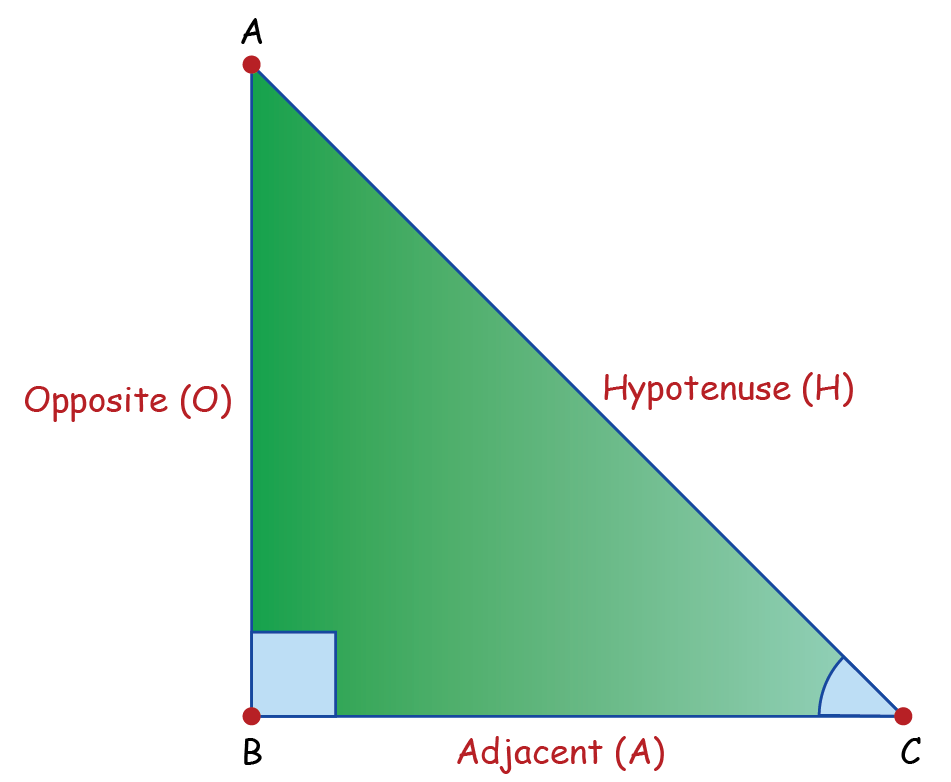# Trigonometry

 1 Introduction to Trigonometry 2 Trigonometry Basics 3 Trigonometric Identities 4 Trigonometric Table 5 Trigonometric Formulae 6 FREE Worksheets of Trigonometry

## Introduction

Trigonometry deals with the measurement of angles and problems related to angles.

The word trigonometry is a 16th century Latin derivative.It is a branch of mathematics which deals with the relation between the angles and sides of a triangle.

You can know about triangles and their different types here.

You can also go ahead and explore all important topics in Trigonometry by selecting the topics from this list below:

Let’s get a better idea of trigonometry with an example.

Rohit is standing near a tree.

He looks up at the tree and wonders “How tall is the tree?”You would be amazed to know that he can find the height of the tree without actually measuring it.

Wonder how?

That’s where trigonometry would help us. The above image can be simplified as below.What we have here is a right-angled triangle, i.e.: a triangle with one of the angles equal to $$90$$ degrees.

The height of the tree can be found out by using basic trigonometric formulae.

## Trigonometric Basics

Trigonometry basics deal with the measurement of angles and problems related to angles. Let’s look at the diagram below.Let’s define a few terms that will be used extensively in trigonometry

 Adjacent It is the side of the triangle which is adjacent to (or below) angle \theta. $$\text{BC}$$ is the adjacent side. Opposite It is the side of the triangle which is opposite to angle \theta. $$\text{AB}$$ is the opposite side. Hypotenuse It is the largest side of the triangle. $$\text{AC}$$ is the hypotenuse. Angle of Elevation It is the angle between the horizontal plane and the line of sight from an observer's eye to an object above. $$\theta$$ is the angle of elevation.

Considering trigonometry has a lot of real-life applications such as measuring precise distances, developing music and more, it becomes necessary to learn about the trigonometric basics.

We will cover a lot of topics in this section like Trigonometric Ratios, Basic Properties of Trigonometric Ratios, Trigonometric ratios of Specific Angles, Trigonometric Elimination and Trigonometric Ratios of Complementary Angles.

We will also explore some of the topics like Heights and Distances, Sine Law, Cosine Law, What is a Radian, Trigonometric Ratios in Radians, Trigonometric Ratios of Arbitrary Angles, Conversion Relations of Trigonometric Ratios, Sine Function, Cosine Function, Tangent Function, Cosecant, Secant & Cotangent Functions, Inverse Trigonometric Ratios. Further on, you will get to learn more about Inverse Trigonometric Ratios and Inverse Trigonometric Ratios for Arbitrary Values.

## Trigonometric Identities

In Trigonometric Identities, an equation is called an identity when it is true for all values of the variables involved. Similarly, an equation involving trigonometric ratios of an angle is called a trigonometric identity, if it is true for all values of the angles involved.

### Example:

 \begin{align} \text{sin } \theta ÷ \text{cos } \theta &= \left[\frac{\text{Opposite}}{\text{Hypotenuse}}\right] ÷\left[\frac{\text{Adjacent}}{\text{Hypotenuse}}\right]\\&= \frac{\text{Opposite}}{\text{Adjacent}}\\&= \text{tan } \theta \end{align}

Therefore, $$\text{tan }\theta = \text{sin }\theta ÷ \text{cos }\theta$$ is a trigonometric identity.

## Trigonometric Table

The trigonometric table is made up of trigonometric ratios that are interrelated to each other – sine, cosine, tangent, cosecant, secant, cotangent. These ratios, in short, are written as sin, cos, tan, cosec, sec and cot.

You can refer to the trigonometric table chart to know more about these ratios.

## Trigonometric Formulae

The complete list of trigonometric formulae involving trigonometric ratios and trigonometric identities is listed for easy access.

Here's a list of all the trigonometric formulas for you to learn and revise.